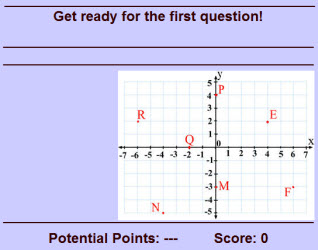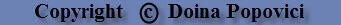# MATH-PLAY.COM## Coordinate Plane Game

In this coordinate plane game, students will have to locate ordered pairs and find the coordinates of various points. The faster you answer, the more points you get.

This game is suitable for 5th grade students, but also for middle school students.

Note: This activity works on computers and laptops, but not iPads or other tablets.This game is based on the following Common Core Math Standards: CCSS.Math.Content.5.G.A.1 Use a pair of perpendicular number lines, called axes, to define a coordinate system, with the intersection of the lines (the origin) arranged to coincide with the 0 on each line and a given point in the plane located by using an ordered pair of numbers, called its coordinates. Understand that the first number indicates how far to travel from the origin in the direction of one axis, and the second number indicates how far to travel in the direction of the second axis, with the convention that the names of the two axes and the coordinates correspond (e.g., x-axis and x-coordinate, y-axis and y-coordinate). CCSS.Math.Content.5.G.A.2 Represent real world and mathematical problems by graphing points in the first quadrant of the coordinate plane, and interpret coordinate values of points in the context of the situation.

Return from the Coordinate Plane Game to Math Matching Games or to Math Play.